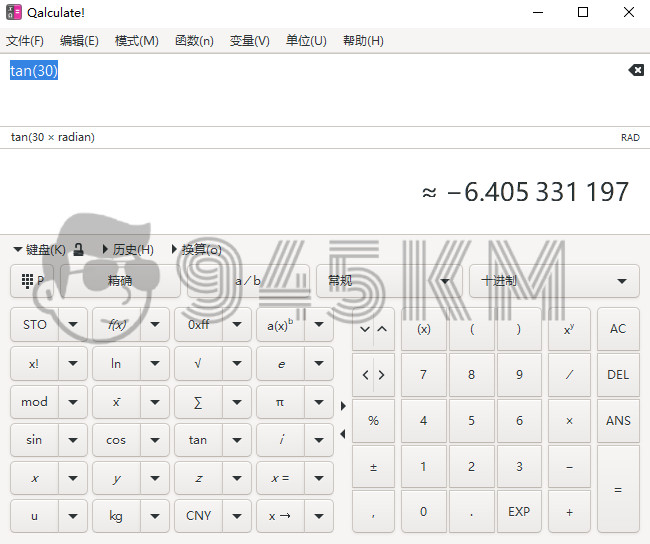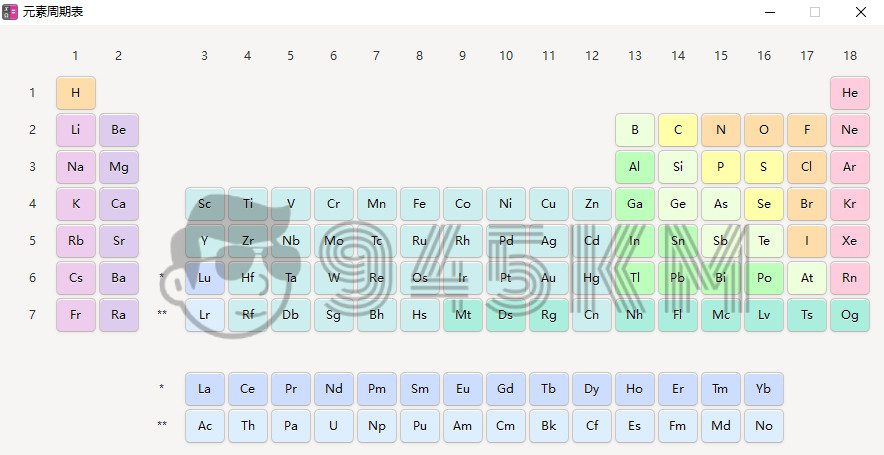# 【Windows】Qalculate v4.10 跨平台、全免费、全汉化的桌面计算器

## 软件介绍

Qalculate，是一款外表简单易用、内核强大且功能丰富的跨平台、全免费的计算器应用，它支持Windows、Linux和MacOS平台。其功能包含普通计算、科学计算、自定义函数、单位、高计算精度、作图以及可以输入一行表达式的图形界面（也可以选择使用传统按钮）。

## 软件截图## 软件特色

https://qalculate.github.io/manual/index.html

#### 数学库

• 计算和解析：
• 基本操作和运算符：+ - * / mod ^ E() && || ！< > >= <= != ~ & | << >>异或
• 字符串的容错解析：log 5 / 2 .5 (3) + (2( 3 +5 = ln(5) / (2.5 * 3) + 2 * (3 + 5))
• 表达式可以包含数字、函数、单位、变量、向量和矩阵以及日期的任意组合
• 支持复数和无限数
• 不确定性的传播
• 区间算术
• 支持所有常见的数基，以及负数和非整数基数、六十进制数、时间格式和罗马数字
• 能够禁用函数、变量、单位或未知变量以减少混淆：例如，当您不希望 (a+b)^2 表示 (are+barn)^2 但 ("a"+"b")^2
• 可定制的隐式乘法
• 矩阵和向量，以及相关运算（行列式等）
• 详细的错误消息
• 任意精度
• RPN模式
• 结果显示：
• 支持所有常见的数基，以及负数和非整数基数、六十进制数、时间格式和罗马数字
• 许多自定义选项：精度、最大/最小小数、复数形式、乘号等。
• 精确或近似：sqrt(32) 返回 4 * sqrt(2) 或 5.66
• 简单分数和带分数：4 / 6 * 2 = 1.333... = 4/3 = 1 + 1/3
• 符号计算：
• 例如 (x + y)^2 = x^2 + 2xy + y^2；4 个“苹果”+ 3 个“橙子”
• 分解和简化
• 差异化与整合
• 可以解大部分方程和不等式
• 可定制的假设给出不同的结果（例如 ln(2x) = ln(2) + ln(x) 如果 x 被假定为正数）
• 职能：
• 数以百计的灵活函数：三角函数、指数和对数、组合、几何、微积分、统计、金融、时间和日期等。
• 可以轻松创建、编辑和保存到标准 XML 文件
• 单位：
• 支持所有 SI 单位和前缀（包括二进制），以及英制和其他单位系统
• 自动换算：ft + yd + m = 2.2192 m
• 显式转换：5 m/s 到 mi/h = 11.18 英里/小时
• 智能换算：5 kg*m/s^2自动换算成5 N
• 通过检索每日汇率进行货币转换
• 不同的名称形式：缩写、单数、复数（米、米、米）
• 可以轻松创建、编辑和保存到标准 XML 文件
• 变量和常量：
• 基本常数：pi、e等。
• 许多物理常数（带或不带单位）和化学元素的性质
• CSV文件导入导出
• 可以轻松创建、编辑和保存到标准 XML 文件
• 灵活 - 可以包含简单的数字、单位或完整的表达式
• 具有类似数据库结构的对象和相关属性的数据集
• 绘图：
• 使用 Gnuplot
• 可以绘制函数或数据（矩阵和向量）
• 能够将绘图保存为 PNG 图像、附言等。
• 几个自定义选项

## 软件下载

• 修改版本安卓软件，加群提示为修改者自留，非本站信息，注意鉴别
• 下载声明：本站部分资源来自于网络收集，若侵犯了你的隐私或版权，请及时联系我们删除有关信息

THE END